# Texas Go Math Grade 7 Lesson 10.2 Answer Key Volume of Triangular Prisms and Pyramids

Refer to our Texas Go Math Grade 7 Answer Key Pdf to score good marks in the exams. Test yourself by practicing the problems from Texas Go Math Grade 7 Lesson 10.2 Answer Key Volume of Triangular Prisms and Pyramids.

## Texas Go Math Grade 7 Lesson 10.2 Answer Key Volume of Triangular Prisms and Pyramids

Essential Question
How do you find the volume of a triangular prism or a triangular pyramid?

Finding the Volume of a Triangular Prism
The volume V of a prism is the area of its base B times its height h, or V = Bh .

Example 1
Find the volume of the triangular prism.
Step 1
Find the area of the triangular base.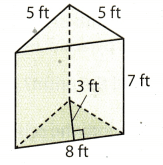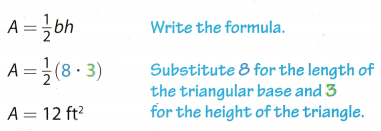Step 2
Find the volume of the triangular prism.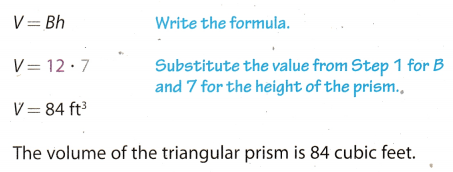Reflect

Question 1.
What If? Suppose you know the volume V and base area B of a triangular prism. How could you find the height of the prism?
raggedright The volume of a triangular prism is:
V = B . h (1)
We know the area of the base B and the volume of a triangular prism.
Divide (1) by B.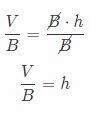Question 2.
Find the volume of a garden seat in the shape of a triangular prism with a height of 30 inches and a base area of 72 in2.
V = B . h
We know the base B and the height of a prism.
B = 72 in2
h = 30 in
Subtitute vaWe of base and height of a prism.
V = 72 . 30
= 2160 in3

The volume of a garden seat is 2160 in3.

Exploring the Volume of a Triangular Pyramid

Previously you explored the volumes of rectangular prisms and pyramids. Now you will repeat the same activity with triangular pyramids and prisms that have the same height and congruent bases.

Step 1
Make three-dimensional models. Make larger versions of the nets shown. Make sure the bases and heights in each net are the same size. Fold each net, and tape it together to form an open prism or pyramid.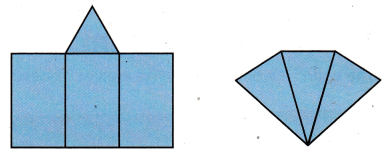Step 2
Fill the pyramid with bears. Make sure that the beans are level with the opening of the pyramid. How many pyramids full of beans do you think
it will take to fill the prism? ________________________
Pour the beans into the prism. Repeat until the prism is full.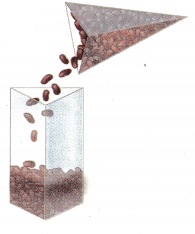Step 3
Write a fraction that compares the volume of the pyramid to the volume of the prism.

Reflect

Question 3.
Analyze Relationships Does it appear that the relationship between the volume of triangular pyramids and prisms is the same as that for rectangular pyramids and prisms?
The volume of the triangular pyramids and prisms with congruent bases and heights are the same with how the
volume of rectangular pyramids and prisms are determined. The relationship between the two are the same in
which the volume of the triangular pyramid is $$\frac{1}{3}$$ the volume of the triangular prism.

Yes

Question 4.
Draw Conclusions Write a formula for the volume of a triangular pyramid with a base area of B and a height of h.
We know that the volume V of a triangular pyramid is the area of its base B times its one-third of height h.
Formula for volume = $$\frac{1}{3}$$Bh
where, B is area of base and h is height of triangular pyramid.

V = $$\frac{1}{3}$$Bh

Question 5.
The volume of a triangular pyramid is 13.5 m3. What is the volume of a triangular prism with a congruent base and the same height? Explain.
ragged right The volume of a triangular prism is
V = B . h (1)
The volume of triangular pyramid is
VP = $$\frac{1}{3}$$B . h (2)
They have same value for base and height.
Multiply (2) by 3
3VP = B . h = V
The volume of a triangular prism is three time as big as the volume of a pyramid.
The volume of a triangular prism is:
V = 3 . VP = 3 . (13.5) = 40.5 m3
The volume of a triangular prism is three time as big as the volume of a pyramid.
The volume of a triangular prism is:
V = 3 VP = 40.5 m3

Example 2
Mr. Martinez is building wooden shapes for a sculpture in the park. His plans show a triangular pyramid and a triangular prism, and each shape is 5 feet high. The base of each shape is a triangle with a base of 2.5 feet and a height of 2 feet. How much greater than the volume of the pyramid is the volume of the prism?Step 1
The triangular base is the same for both shapes. Find the area of the base B.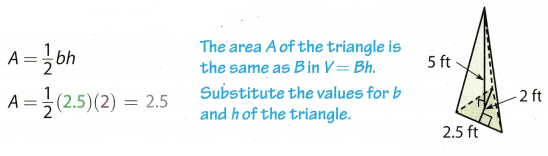The area of the base B for both shapes is 2.5 ft2.

Step 2
Find the volume of the prism.
V = Bh Use the formula.
V = (2.5) × 5 = 12.5 Substitute the value for B and h of the prism.
The volume of the prism is 12.5 ft3.

Step 3
Find the volume of the pyramid.
Volume of pyramid = $$\frac{1}{3}$$ . volume of prism
= $$\frac{1}{3}$$ . 12.5 ≈ 4.2 Substitute and calculate.
The volume of the pyramid is approximately 4.2 ft3.

Step 4
Compare the volumes.
Volume of prism – volume of pyramid = 12.5 – 4.2 = 8.3
The volume of the prism is 8.3 ft3 greater than that of the pyramid.

Question 6.
How much greater is the volume of a triangular prism with base area of 14 cm2 and height of 4.8 cm than the volume of a triangular pyramid with the same height and base area?
Use formula for a prism.
V = B . h
B = 14 cm2 Base area of a prism
h = 4.8 cm Height of a prism
y = 14 . (4.8)
V = 67.2 cm3 The volume of a prism
Use formula for a pyramid.
V = $$\frac{1}{3}$$ . B . h = $$\frac{1}{3}$$ . volume of a prism
= $$\frac{1}{3}$$ . (67.2)
V = 22.4
Volume of a prism – volume of a pyramid = 44.8 cm3
The volume of a prism is 44.8 cm3 greater than that of the pyramid.

Question 1.
Find the volume of the triangular prism. (Example 1)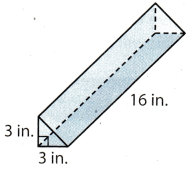Find the area of the base of the prism.
Use the equation A = ______ bh.
A = ___ (___) (___) = ___ in2
Find the volume of the prism. Use the equation V = Bh.
V = (___)(___) = ___ in3
b = 3 in Length of base
h = 3 in Height of base
h = 16 in Height of a prism
A = $$\frac{1}{2}$$ . b. h
A = $$\frac{1}{2}$$ . 3 . 3 = $$\frac{9}{2}$$ = 4.5 in2
V = B . h
V = (4.5) . 16 = 72 in3
V = 72 in3

Question 2.
A triangular pyramid and a triangular prism have congruent bases and the same height. The triangular pyramid has a volume of 90 m3. Find the volume of the prism. (Explore Activity)
The volume of the prism is ___ because the volume of the prism is ___ times the volume of the pyramid.
The volume of a prism is:
V = B . h
The volume of a pyramid is:
VP = $$\frac{1}{3}$$ . B . h
They have congruent bases and the same height so the volume of the prism is:The volume of the prism is
270 m3
because the volume of the prism is three times the volume of the pyramid.

Question 3.
In Exercise 2, how much greater is the volume of the prism than the volume of the pyramid? (Example 2)
The volume of the pyramid is only of the volume of the prism. Since the volume of the prism is 270 m3 and the volume of the pyramid is 90 m3. The volume of the prism is greater than the volume of the pyramid by 180 m3

Find the volume of each figure.

Question 4.b = 9 ft Lenghtofthe base
H = 5 ft Height of a prism
h = 9 ft Height of the base
Find the area of the base B We have triangular base, so we use the formula:
B = $$\frac{1}{2}$$ . b . h = $$\frac{1}{2}$$ . 9 . 9 = $$\frac{81}{2}$$ = 40.5 ft2
Use the formula for the voLume of a prism.
V = B . H= (40.5) . 5 = 202.5 ft3

V = 202.5 ft3

Question 5.l = 6ft Lenght of the base
w = 6 ft Width of the base
h = 6 ft Height of a pyramid
Find the area of the base B.
B = l . w = 6 . 6 = 36 ft2
Use tne formula.
V = $$\frac{1}{3}$$ . B . h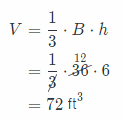V = 72 ft3

Question 6.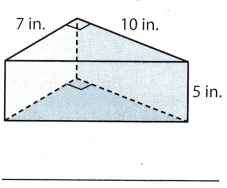a = 7 in The leg of a right triangle
b = 10 in The leg of a right triangle
h = 5 in Height of a prism
Find the area of the base B.Use the formula.
V = B . h = 35.5 = 175 in3

V = 175 in3

Essential Question Check-In

Question 7.
A pyramid has a base that is a triangle. The length of the base of the triangle is 5 meters, and the height of the triangle is 12 meters. The height of the pyramid is 10 meters. How would you explain to a friend how to find the volume of the pyramid?
b = 5 m Length of base
hB = 12 m Height of base
h = 16 m Height of a pyramid
Find the area of the base B. The base is in the shape of triangle. Use the formula:Use the formula for the volume of a triangular pyramid: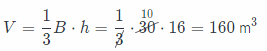The volume of the triangular pyramid is 160 m3.

Question 8.
A trap for insects is in the shape of a triangular prism. The area of the base is 3.5 in2 and the height of the prism is 5 in. What is the volume of this trap?
A trap for insect is in the shape of triangular prism, so use the formula for the volume of a triangular prism.
B = 3.5 in2 Base of a prism
h = 5 in Height of a prism
The volume of a prism is
V = Bh = (3.5) . 5 = 17.5 in3
The volume of a trap for insect is 17.5 in3.

Question 9.
Arletta built a, cardboard ramp for her little brothers’ toy cars. Identify the shape of the ramp. Then find its volume.A cardboard ramp is in the shape of a triangular prism. Use the formula for the volume of a triangular prism.
a = 25 in Length of one side of the triangle
b = 6 in Length of other side of the triangle
h = 7 in Height of a prism
Find the area of the base B.The volume of a triangular prism is:
V = B . h = 75 . 5 = 525 in3

The volume of a cardboard ramp is 525 in3.

Question 10.
Represent Real-World Problems Sandy builds this shape of four congruent triangles using clay and toothpicks. The area of each triangle is 17.6 cm2, and the height of the shape is 5.2 cm. What three-dimensional
figure does the shape Sandy built resemble? If this were a solid shape, what would be its volume? Round your answer to the nearest teñth.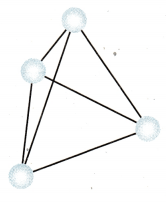Sandy built a three-dimensional figure which resemble on the triangular pyramid
B = 17.6 cm2 Base of pyramid
h = 5.2 cm Height of a pyramid
Use the formula for the volume of a pyramid.
V = $$\frac{1}{3}$$ . 17.6 . 5.2
= 30.50 ≈ 31 cm3

Sandy built a three-dimensional figure which resemble on the triangular pyramid.
The volume of the triangular pyramid is 31 cm3.

Question 11.
Draw Conclusions Would tripling the height of a triangular prism triple its volume? Explain.
B = B Base area
h = h Height of a prism
Use the formula for the volume of a triangular prism.
V1 = Bh
Now triple the height of a prism.
B = B Base area
h = 3 . h Height of a prism
Use the formula for the volume of a triangular prism.
V2 = B . 3 . h = 3 (B . h) = 3 . V1

Tripling height of a triangular prism triples its volume.

Question 12.
The Jacksons went camping in a state park. One of the tents they took is shown. What is the volume of the tent?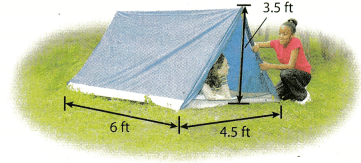b = 4.5 ft Length of base
hB = 3.5 ft Height of base
h = 6ft Height of a prism
Find the area of the base B. The tent is in the shape of a triangular prism. A prism has a base that is triangle, so use the formula:
B = $$\frac{1}{2}$$ . b . hB = $$\frac{1}{2}$$(4.5) . (3.5) = 7.875 ft2
The volume of a triangular prism is:
V = B . h = (7.875) . 6 = 47.25 ft3
The volume of the tent is 47.25 ft3.

Question 13.
Shawntelle is solving a problem involving a triangular pyramid. You hear her say that bee” is equal to 24 inches. How can you tell if she is talking about the base area B of the pyramid or about the base b of the triangle?
Shawntelle while solving a problem she say ‘bee’ is equal to 24 inches. So she is definitely talking about the base
b of the triangle not the base area B. Because she use the unit of base as inches not inch2. If she was taking about the base area then she would have must mentioned the unit as inch2 inches of inches.
Hence, she is talking about the base b of the triangle not base area B.

Question 14.
Alex made a sketch for a homemade soccer goal he plans to build. The goal will be in the shape of a triangular prism. The legs of the right triangles at the sides of his goal measure 4 ft and 8 ft, and the opening along the front is 24 ft. How much space is contained within this goal?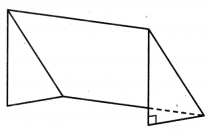b = 4 ft Length of base
hB = 8 ft Height of base
h = 24 ft Height of a prism
Find the area of the base B. The base is in the shape of triangle. Use the formula:Use the formula for the volume of a triangular prism.
V = B . h = 16 . 24 = 384 ft3
This goal contained 384 ft3 of space.

Texas Go Math Grade 7 Lesson 10.2 Higher Order Thinking Answer Key

Question 15.
A plastic puzzle in the shape of a triangular prism has bases that are equilateral triangles with side lengths of 4 inches and a height of 3.5 inches. The height of the prism is 5 inches. Find the volume of the prism.
b = 4 in Length of base
hB = 3.5 in Height of base
h = 5 in Height of a prism
Find the area of the base B. The base is in the shape of triangle. Use the formula: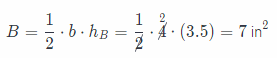Use the formula for the volume of a triangular prism.
V = B . h
= 7 . 5
= 35 in3

The volume of the prism is V = 35 in3.

Question 16.
Persevere in Problem Solving Lynette’s grandmother has a metal doorstop with the dimensions shown. Find the volume of the metal in the doorstop. The metal in the doorstop has a mass of about 8.6 grams per cubic centimeter. Find the mass of the doorstop.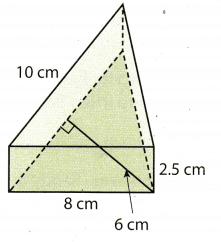b = 10 cm Length of base
hB= 6 cm Height of base
h = 2.5 cm Height of a prism
Find the area of the base B. The base in the shape of triangle. Use the formula:Use the formula for the volume of a triangular prism.
V = B . h = 30 . (2.5) = 75 cm3
Mass of the doorstep is 75 . (8.6) = 645$$\frac{\mathrm{g}}{\mathrm{cm}^{3}}$$.

The volume of the metal in the door step is V = 75 cm3.
Mass of the doorstep is 645$$\frac{\mathrm{g}}{\mathrm{cm}^{3}}$$.

Question 17.
Make a Conjecture Don and Kayla each draw a triangular pyramid that has a volume of 100 cm3. They do not draw identical shapes. Give a set of possible dimensions for each pyramid.
Since Don and Kayta did not draw identical shapes of a triangular pyramid. The possible dimensions for the
triangular pyramid with volume of 100 cm3 are:

• base = 15 cm; height = 10 cm; Height = 4 cm
• base = 10 cm; height = 12 cm; Height = 5 cm
• base = 12 cm; height = 5 cm; Height = 10 cm
• base = 5 cm; height = 10 cm; Height = 12 cm

The possible dimensions for the triangular pyramid with volume of 100 cm3.

Question 18.
Multistep Don’s favorite cheese snack comes in a box of six pieces. Each piece of cheese has the shape of a triangular prism that is 2 cm high. The triangular base of the prism has a height of 5 cm and a base of 4 cm. Find the volume of cheese in the box.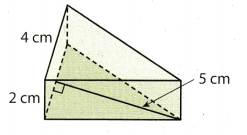Each piece of cheese has the shape of a triangular prism.
For one piece the volume is:
b = 4 cm Length of base
hB = 5 cm Height of base
h = 2 cm Height of a prism
Find the area of the base B.Use the formula for the volume of a triangular prism.
Vc = B . h = 10 . 2 = 20 cm3
We have six pieces in the box, so the volume of cheese in the box is:
V = 6 . Vc = 6 . 20 = 120 cm3

The volume of cheese in the box is V= 120 cm3.

Question 19.
Analyze Relationships What effect would doubling all the dimensions of a triangular pyramid have on the volume of the pyramid? Explain your reasoning.
B = B Base area
h = h Height of a pyramid
Use the formula for the volume of a triangular pyramid.
V1 = $$\frac{1}{3}$$ . B . h
Now double all the dimension of the triangular pyramid.
B = 2 . B Base area
h = 2 . h Height of a pyramid
Use the formula for the volume of a triangular pyramid.
V2 = $$\frac{1}{3}$$ . 2 . B . 2 . h
= $$\frac{1}{3}$$ . 4 . (B . h)
= 4 . $$\frac{1}{3}$$ . (B . h)
= 4 . V1
Doubting at the dimension of a triangular pyramid, its volume is quadrupling

Scroll to Top